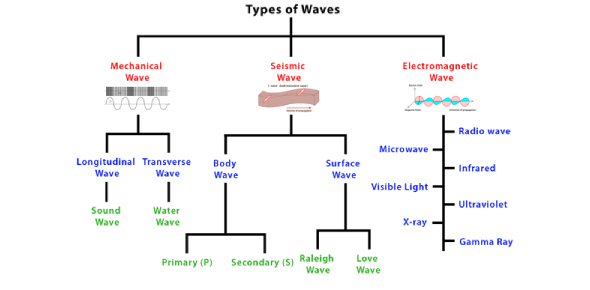# Introduction To Waves Types! Physics Quiz

10 Questions | Total Attempts: 699SettingsA wave transmits information or energy from one point to another. Waves are mainly divided into mechanical, electromagnetic, and matter waves. These three types are differentiated based on how they are made and transmitted. Do you know what a wave trough is or how to get the wavelength? This quiz is designed to help you refresh your basic understanding of waves. Give it a try!

• 1.
The wavelength is represented by which letter?
• A.

A

• B.

B

• C.

C

• D.

D

• E.

E

• 2.
The wave crest is represented by which letter?
• A.

A

• B.

B

• C.

C

• D.

D

• E.

E

• 3.
The wave trough is represented by which letter?
• A.

A

• B.

B

• C.

C

• D.

D

• E.

E

• 4.
What is it called when a certain number of waves pass a given point per a certain number of seconds?
• A.

Frequency

• B.

Amplitude

• C.

Wavelength

• D.

Origin

• E.

Trough

• 5.
What is another name for wave height?
• A.

Amplitude

• B.

Wavelength

• C.

Frequency

• D.

Crest

• E.

Trough

• 6.
The origin or the undisturbed position of the wave is represented by which letter?
• A.

B

• B.

D

• C.

A

• D.

E

• E.

C

• 7.
Which letter represents amplitude?
• A.

B

• B.

A

• C.

D

• D.

E

• E.

C

• 8.
What is measured from one wave crest to another wave crest or one wave trough to another wave trough?
• A.

Crest

• B.

Trough

• C.

Wavelength

• D.

Amplitude

• E.

Frequency

• 9.
What waves have movement that is perpendicular to the direction of the wave?
• 10.
Which type of wave has movement that is parallel to the direction of the wave?
• A.

Transverse

• B.

Compressional or longitudinal

Related TopicsBack to top